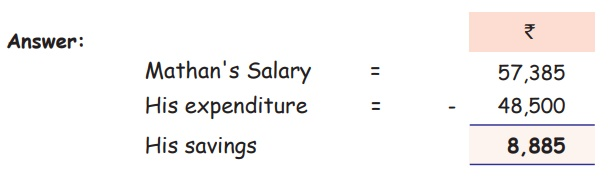Home | | Maths 5th Std | Subtraction

# Subtraction

The result of subtracting one number from another is called the Difference.

Numbers and Operations

Appreciate the role of place value in addition, subtraction and multiplication algorithm

Subtraction

We have already learnt how to add the numbers and to write down the numbers with their corresponding place values. In the same manner we have to do subtraction problem. The operation or process of finding the difference between two numbers or quantities, denoted by a minus sign (−).

The result of subtracting one number from another is called the DifferenceExample

Mathan is working in a construction company. He is getting 57,385 salary per month. He spent 48,500 for his family every month. how much does he save per month?Tags : Numbers | Term 1 Chapter 2 | 5th Maths , 5th Maths : Term 1 Unit 2 : Numbers
Study Material, Lecturing Notes, Assignment, Reference, Wiki description explanation, brief detail
5th Maths : Term 1 Unit 2 : Numbers : Subtraction | Numbers | Term 1 Chapter 2 | 5th Maths